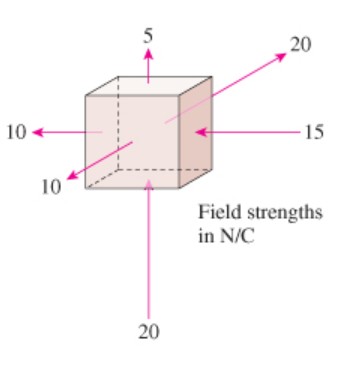# Problem: The electric field is constant over each face of the cube shown in the figure (Figure 1) .Does the box contain positive charge, negative charge, or no charge?

###### FREE Expert Solution

Gauss' law:

$\overline{){\mathbf{\varphi }}{\mathbf{=}}{\mathbf{\oint }}{\mathbf{E}}{\mathbf{·}}{\mathbf{d}}{\mathbf{A}}{\mathbf{=}}\frac{{\mathbf{q}}_{\mathbf{e}\mathbf{n}\mathbf{c}\mathbf{l}\mathbf{o}\mathbf{s}\mathbf{e}\mathbf{d}}}{{\mathbf{\epsilon }}_{\mathbf{0}}}}$

Take field out of the box as positive and field into the box as negative, because electric field originates from a positive charge and ends at a negative charge.

99% (161 ratings)###### Problem Details

The electric field is constant over each face of the cube shown in the figure (Figure 1) .Does the box contain positive charge, negative charge, or no charge?